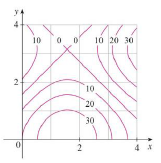Chapter 15.1, Problem 7E

Chapter
Section
Textbook Problem

A contour map is shown for a function f on the square R = [0, 4] × [0. 4].(a) Use the Midpoint Rule with m = n = 2 to estimate the value of ∬ R f ( x , y ) d A .(b) Estimate the average value of f.(a)

To determine

To estimate: The value of given double integral over the rectangle R by using midpoint rule.

Explanation

Formula used:

The double integral, Rf(x,y)dAi=1mj=1nf(x¯i,y¯j)ΔA ,

Where, ΔA=a2 , where a is the side of each square.

The given function is f(x,y) .

The mid points of each rectangle is denoted by (x¯i,y¯j) , where x¯i is the midpoint of [xi1,xi] and y¯i is the midpoint of [yj1,yj] .

The Riemann sum constants are denoted by m, n.

Given:

The rectangular region, R=[0,4]×[0,4] .

The Riemann sum constants, m=2,n=2 .

Calculation:

Plot the given rectangle in the graph and pick the mid points of each rectangle.

From Figure 1, it is observed that the mid points of each rectangle is (1,1),(1,3),(3,1)(3,3) and a=2

(b)

To determine

To estimate: The average value of given function.

Still sussing out bartleby?

Check out a sample textbook solution.

See a sample solution

The Solution to Your Study Problems

Bartleby provides explanations to thousands of textbook problems written by our experts, many with advanced degrees!

Get Started

Use the given graph of f to sketch the graph of f1. 29.

Single Variable Calculus: Early Transcendentals, Volume I

In Exercises 75-98, perform the indicated operations and/or simplify each expression. 88. (3a 4b)2

Applied Calculus for the Managerial, Life, and Social Sciences: A Brief Approach

limx(12)x2= a) 0 b) 1 c) d) does not exist

Study Guide for Stewart's Single Variable Calculus: Early Transcendentals, 8th